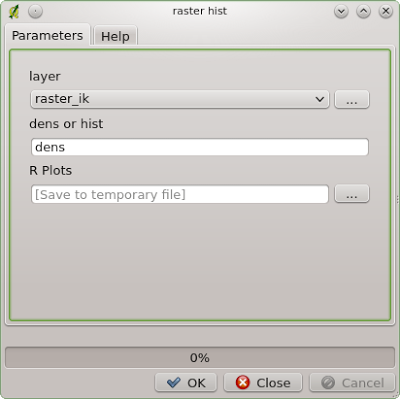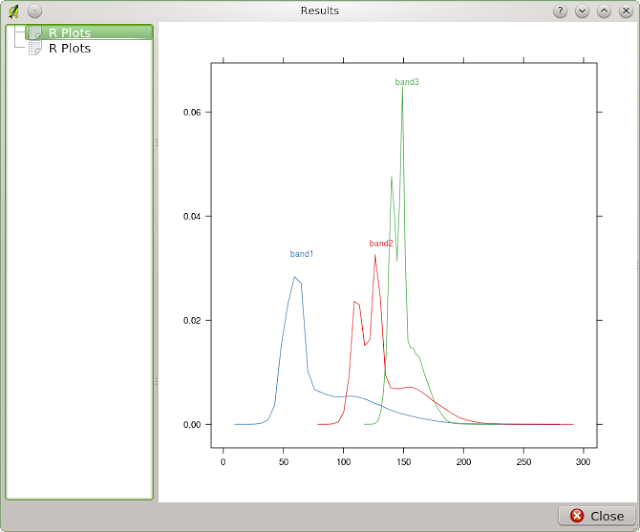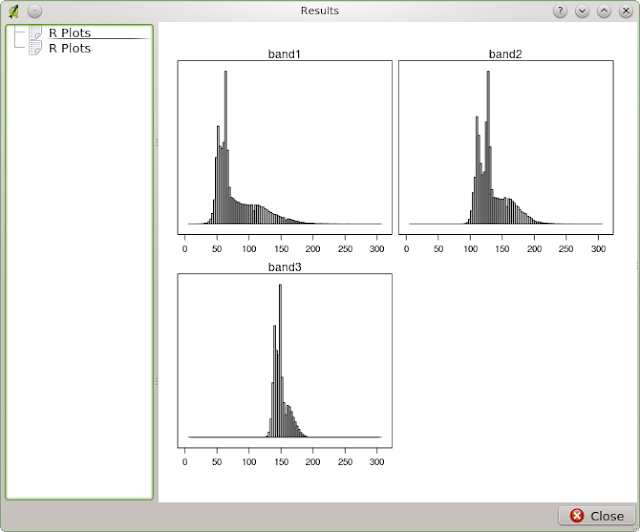Misanthrope's Thoughts

What is the cause for the degradation of environment?
Capitalism, corruption, consuming society? - OVERPOPULATION!
Please, save the Planet - kill yourself...

Introduction

This us the first post of my series notes about hyperspectral imagery classification. See other parts: Part 2: Classification Approach and Spectral Profiles Creation
There was the EEEI Data Fusion Contest this spring. This year they wanted people to elaborate about hyper-spectral (142-bands imagery) and LiDAR data. The resolution of the data-set was about 5 m.  There were 2 nominations: best classification and  the best scientific paper.

I work with high-resolution imagery quite often, but classification is a very rear task for me though. I thought that this contest was a great opportunity to develop my skills. And not just a classification skills, but R skills as well... I decided to participate in best classification contest, and to use R for the most part.

I learned a lot and I will share my knowledge with you in a series of posts starting with this one. And like in some great novels, I will start from the very end - evaluation of my results.Results of my classification (created in R, designed in QGIS)

Contest results

...are available here. As you may notice, I'm not on the list of the 10 best classification ;-) But there is almost unnoticeable 0.03% difference between my result (85.93% accuracy) and the result of the 10-th place. Not a bad result for a relatively inexperienced guy, don't you think? And I know, that I could have done better - I had 99% prediction accuracy for the training samples. It's funny, but my classification map looks better than map that took 7-th place!

How to evaluate classification using R

Due to I was not on the top ten list, I had to evaluate the result on my own. The organisers finally disclosed evaluation samples and I got a chance for a self assessment. So we have a set of .shp-files - each contains ground-truth polygons for one of the 16 classes and a classification map. We need to accomplish 3 goals:
1. Create a visual representation of missclassification.
2. Assess accuracy.
3. Create a confusion matrix.
4. Visualise classification map using EEEI colour palette.

Lets get a palette!Official EEEI palette
To extract colour values from the palette above you may use GIMP. But I used a widget that every KDE-user (Linux) should have by default. You can probe and save colour values from any part of your screen. Quite useful!'Color picker' in work
Now let's see the code for our tasks.

library(rgdal)
library(raster)
library(reshape2)
library(caret)
library(ggplot2)

Load and process data

# get classification raster
verbose = T)
# get list of shp-files for evaluation
shapes <- list.files(path = '~/GIS/IEEE_contest_2013/2013_IEEE_GRSS_DF_Contest/roi', pattern = '*shp')
# a list for accuracy assessment
accuracy_list <- list()

# create an empty dataframe to be filled vith evaluation results
# field names are not arbitrary!!!
eval_df <- data.frame(variable = character(),
value = character())

# create an empty dataframe to be used for plotting
# field names are not arbitrary!!!
plot_data <- data.frame(variable = character(),
value = character(),
Freq = integer())

for (f_name in shapes) {
# delete '.shp' from the filename
layer_name <- paste(sub('.shp', '', f_name))
layer = layer_name,
verbose = F)

# extract values from raster
probe <- extract(ras, class)

# replace class numbers with names
samples <- list()
for (lis in probe) {
for (value in lis) {
if (value == 0) {c_name <- Unclassified
} else if (value == 1) {c_name <- 'Healthy grass'
} else if (value == 2) {c_name <- 'Stressed grass'
} else if (value == 3) {c_name <- 'Synthetic grass'
} else if (value == 4) {c_name <- 'Trees'
} else if (value == 5) {c_name <- 'Soil'
} else if (value == 6) {c_name <- 'Water'
} else if (value == 7) {c_name <- 'Residential'
} else if (value == 8) {c_name <- 'Commercial'
} else if (value == 9) {c_name <- 'Road'
} else if (value == 10) {c_name <- 'Highway'
} else if (value == 11) {c_name <- 'Railway'
} else if (value == 12) {c_name <- 'Parking Lot 1'
} else if (value == 13) {c_name <- 'Parking Lot 2'
} else if (value == 14) {c_name <- 'Tennis Court'
} else if (value == 15) {c_name <- 'Running Track'}
samples <- c(samples, c = c_name)
}
}

# make layer_name match sample name
if (layer_name == 'grass_healthy') {layer_name <- 'Healthy grass'
} else if (layer_name == 'grass_stressed') {layer_name <- 'Stressed grass'
} else if (layer_name == 'grass_syntethic') {layer_name <- 'Synthetic grass'
} else if (layer_name == 'tree') {layer_name <- 'Trees'
} else if (layer_name == 'soil') {layer_name <- 'Soil'
} else if (layer_name == 'water') {layer_name <- 'Water'
} else if (layer_name == 'residental') {layer_name <- 'Residential'
} else if (layer_name == 'commercial') {layer_name <- 'Commercial'
} else if (layer_name == 'road') {layer_name <- 'Road'
} else if (layer_name == 'highway') {layer_name <- 'Highway'
} else if (layer_name == 'railway') {layer_name <- 'Railway'
} else if (layer_name == 'parking_lot1') {layer_name <- 'Parking Lot 1'
} else if (layer_name == 'parking_lot2') {layer_name <- 'Parking Lot 2'
} else if (layer_name == 'tennis_court') {layer_name <- 'Tennis Court'
} else if (layer_name == 'running_track') {layer_name <- 'Running Track'}

# create a dataframe with classification results
df <- as.data.frame(samples)
dfm <- melt(df, id = 0)
dfm['variable'] <- layer_name

# add data to evaluation dataframe
eval_df <- rbind(eval_df, dfm)

# assess accuracy of current class
mytable <- table(dfm)
dmt <- as.data.frame(mytable)
total_samples <- 0
correct_predictions <- 0
for (i in 1:nrow(dmt)) {
predict_class <- toString(dmt[i,2])
pc_frequency <- dmt[i,3]
if (predict_class == layer_name) {
correct_predictions <- dmt[i,3]
}
total_samples <- total_samples + pc_frequency
}
accuracy <- round(correct_predictions/total_samples, 2)
accuracy_list <- c(accuracy_list, c = accuracy)

# append data for plotting
plot_data <- rbind(plot_data, dmt)
}

Create a fancy graph (that is shown on the map)

# create facets plot
EEEI_palette <- c('#D4D4F6',
'#5F5F5F',
'#710100',
'#00B300',
'#007900',
'#014400',
'#008744',
'#D0B183',
'#BAB363',
'#DAB179',
'#737373',
'#A7790A',
'#00EA01',
'#CA1236',
'#00B9BB')
plot_data <- plot_data[order(plot_data\$variable),]
p = ggplot(data = plot_data,
aes(x = factor(1),
y = Freq,
fill = factor(value)
)
)
p <- p + facet_grid(facets = . ~ variable)
p <- p + geom_bar(width = 1) +
xlab('Classes') +
ylab('Classification rates') +
guides(fill=guide_legend(title="Classes"))+
scale_fill_manual(values = EEEI_palette)+
ggtitle('Classification Accuracy')+
theme(axis.text.x = element_blank(), axis.ticks.x = element_blank())
p

Let't finally get our accuracy result!

# accuracy assessment
fin_accuracy <- mean(unlist(accuracy_list))
fin_accuracy <- paste(round(fin_accuracy*100, 2), '%', sep = '')
print(paste('Total accuracy:', fin_accuracy), sep = ' ')

 "Total accuracy: 85.93%"

Confusion matrix

# confusion matrix creation
true <- as.factor(eval_df\$variable)
predict <- as.factor(eval_df\$value)
confusionMatrix(predict, true)

Thursday, July 25, 2013

A Note About proj4 in R

It's been a long time since I had to transform some coordinates 'manually'. I mean I had a list of coordinates that I needed to [re]project. There are some nice proprietary tools for it, but who needs them when the opensource is everywhere?

Proj4 is an obvious tool for this task. And using it from within R is somewhat a natural approach when you need to process a csv-file. There is a package called... 'proj4' that provides a simple interface to proj4. It has only two functions: project and ptransform. The first one is meant to provide transition between unprojected and projected coordinate reference systems and the second - between different projected coordinate reference systems.

Look closely at description of the project function: "Projection of lat/long coordinates or its inverse". Ok... here is a huge setup which made me write this post. If you would try to use coordinates in lat/long format here you would screw up! Your points would fly thousands of miles away from initial position. Actually you have to use coordinates in long/lat format to get the result you need. Don't trust manuals ;-)

Oh, I really hate the guy who started this practice of placing longitude before latitude in GIS...

Sunday, July 7, 2013

Getting raster histogram in QGIS using SEXTANTE and R

The issue with the broken histogram creation tool in QGIS annoyed me far too long. Sometimes you just need a quick glance on the histogram of a raster just to make a decision on how to process it or just to assess distribution of classes. But as you know, QGIS histogram tool creates broken plots: they are just useless (don't know if something have changed in Master).

Fortunately there is the SEXTANTE - extremely useful plugin that provides access to hundreds of geoprocessing algorithms and allows to create your own very quickly. SEXTANTE grants access to R functions, and one of the out-of-the-box example scripts, surprise-surprise, is about raster histogram creation. "Hooray! The problem is solved!" - one may cry out. Unfortunately it's not. "Raster histogram" tool is somewhat suitable only for single-band rasters (its algorithm is as simple as hist(as.matrix(raster))). Obviously we need to create our own tool. Luckily it is extremely simple if one is already familiar with R.

To create your own R tool for QGIS go to Analisys menu and activate SEXTANTE toolbox. Then go to R-scripts -> Tools and double-click 'Create new R script' and just copy and paste this code (an explanatoin will follow the description):
UPD: now this code works for both multi-band and single-band rasters. Here is the code for QGIS 1.8.
##layer=raster
##Tools=group
##dens_or_hist=string hist
##showplots
library('rpanel')
library('rasterVis')
str <- dens_or_hist
if (str !='dens' & str != 'hist'){
rp.messagebox('you must enter "dens" or "hist"', title = 'oops!')
} else {
im <- stack(layer)
test <- try(nbands(im))
if (class(test) == 'try-error') {im <- raster(layer)}
if (str == 'dens') {
densityplot(im)
} else if (str == 'hist') {
histogram(im)
}
}

UPD2: Here is the code for QGIS 2.0:
##layer=raster
##Raster processing=group
##dens_or_hist=string hist
##showplots
library('rpanel')
library('rasterVis')
str <- dens_or_hist
if (str !='dens' & str != 'hist'){
rp.messagebox('you must enter "dens" or "hist"', title = 'oops!')
} else {
if (nbands(layer) == 1) {
layer <- as.matrix(layer)
layer <- raster(layer)
}
if (str == 'dens') {
densityplot(layer)
} else if (str == 'hist') {
histogram(layer)
}
}
Now save the script. It will appear among other R scripts. Launch it and you will see this window:Script window
In 'layer' drop-down menu choose needed raster. In 'dens or hist' field type dens or hist to identify which representation of the raster cell data you prefer: density plot or a histogram. [ It would be great to know if it is possible to embed these options in drop-down menu as well (help me if you know how). ] In 'R plots' field set the destination of the graph to be saved (optional). If you chose density plot you will get something like this:Density plot
If you choose histogram, you will get something like this:Histogram
And if you fail to provide correct value in 'dens or hist' field you will get this:Fail window

How it works.

##layer=raster  provides us with the drop-down menu to select needed raster and it will be addresed as layer in our script. ##Tools=group will assign our script to the Tools group of R scripts in SEXTANTE. ##dens_or_hist=string provides us with the string input field dens or hist. ##showplots will show the plot in Results window after execution. library('rpanel') this library is needed to show pop-up window with the error when the user fails to type 4 letters correctly. library('rasterVis') this library works on top of raster package and it is responsible for plotting our graphs. Then we define condition to pop up error window via rp.messagebox if the plot type is misspelled or missing. The following piece of code is interesting:
im <- stack(layer)
test <- try(nbands(im))
if (class(test) == 'try-error') {im <- raster(layer)}
In order to correctely process the raster we need to have a RasterStack class for multi-band raster or a RasterLayer class if the raster is single-banded. 'raster' package is a bit weird with its classes so there are even different functions for determination of the number of bands for different classes and types of rasters. So the code above is weird too))) We assume that the raster is multi-banded and create a RasterStack object. Then we check whether our guess was correct: if raster is actually single-banded we will have an error if we try to use nbands() function on it.  If it is the case - we will create RasterLayer object instead of RasterStack.
Finally, we transform our raster to the RasterStack object to be able to run either densityplot() or histogram() function.

Final notes

In a blink of an eye we were able to create quite handy tool to replace a long broken one. But there is the downside. This script won't be able to handle huge rasters - I wasn't able to process my IKONOS imagery where sides of the rasters exceeds 10000 pixels. Also I would not recommend to use in on a hyperspectral imagery with the dozens of bands because the process will run extremely sloooow (it will take hours and tons of RAM) and the result will be obviously unreadable.